• 导入pandas之前要导入numpy
千次阅读
2021-12-31 21:10:28

## 一、报错描述

由于本人安装Senta库后导致之后python导入pandas包时出现“numpy.ufunc size changed, may indicate binary incompatibility”错误。
具体报错：ValueError: numpy.ufunc size changed, may indicate binary incompatibility. Expected 216 from C header, got 192 from PyObject.

## 二、问题分析

出现这种报错的直接原因是numpy与pandas版本不匹配。

## 三、解决方案

1. 网上许多方案都是让先卸载再重新安装numpy或升级numpy，但是重新安装或更新之后发现还是出现一样的错误。（这个方法不管用）
2. 先卸掉已经安装的numpy库，然后再更新pandas库。（本人成功解决的方法）
pip uninstall numpy
pip install pandaspython
更多相关内容
• 俩个方法在pycharm中导入pandasnumpy，matplotlib这三个包

俩种方法

注：这里使用的是python3.7的版本，如果是3.9版本将pip变为pip3即可，否则会出现版本字符差异导致报错（例如：pip3 install numpy  -i https://pypi.tuna.tsinghua.edu.cn/simple（后面是清华的镜像，会快一些））

方法一：.首先windows + R 打开小黑盒子，输入pip install numpy, pip install pandas ,pip install matplotlib,如果这三个命令可以直接成功，只需要重新打开pycharm---file---Settings---Project:project ---Python interpreter 查看当前的版本是否健在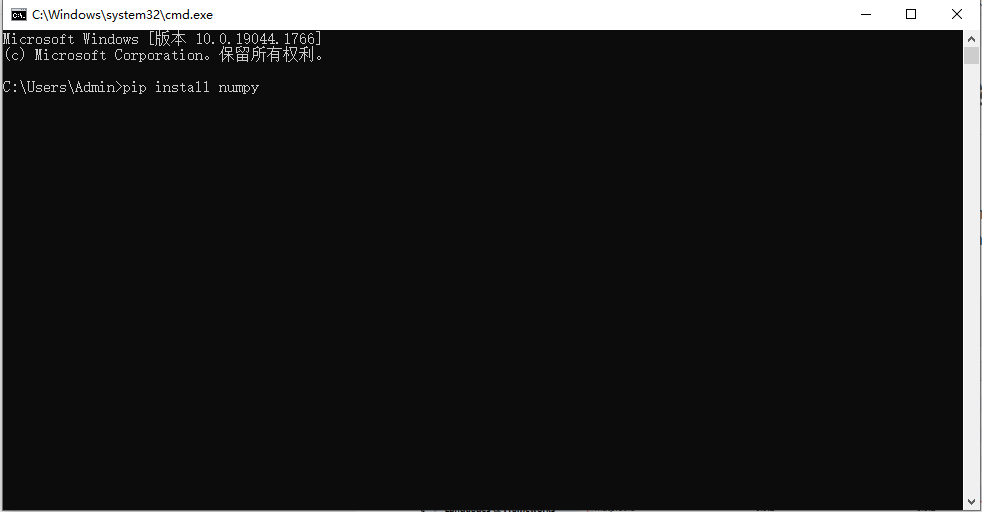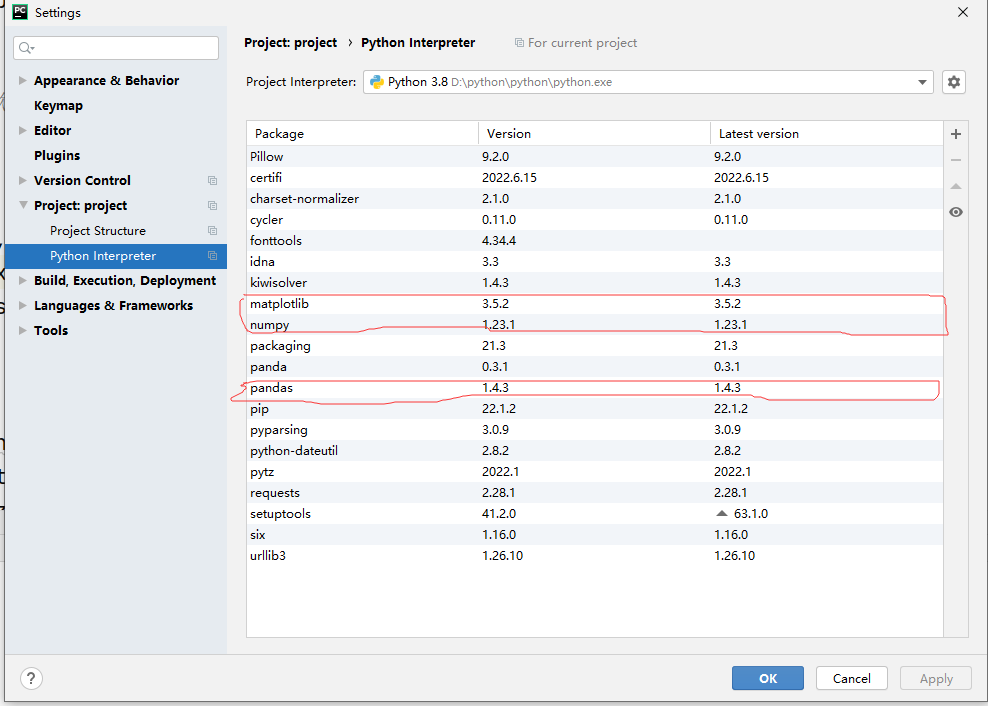上面方法可能会存在的问题：

(1) 执行pip install指令时显示pip的版本不够，需要更新pip的版本，可以在小黑盒子中对pip进行更新，命令为：python -m pip install --upgrade pip

(2)如果上述方法还是更新pip失败

1）卸载pip，输入命令行: python -m pip uninstall pip
2）安装pip，在python文件下的Scripts目录下，打开cmd，输入命令行：easy_install.exe pip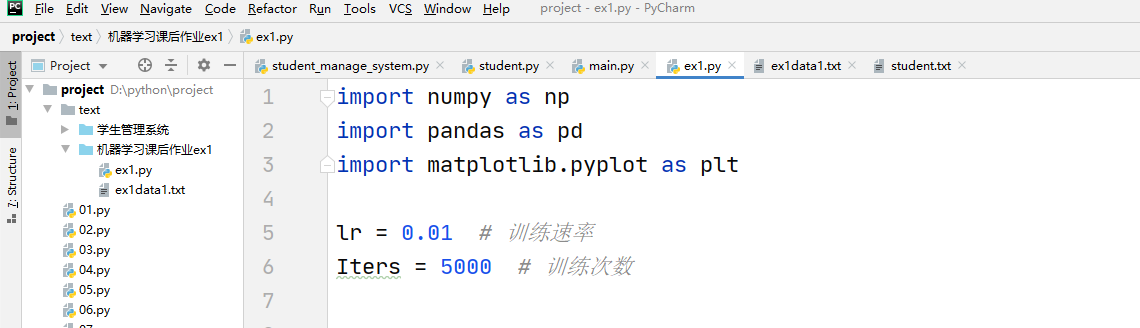这样就安装成功了

方法二：打开pycharm---file---Settings---Project:project ---Python interpreter，点击右上角的+号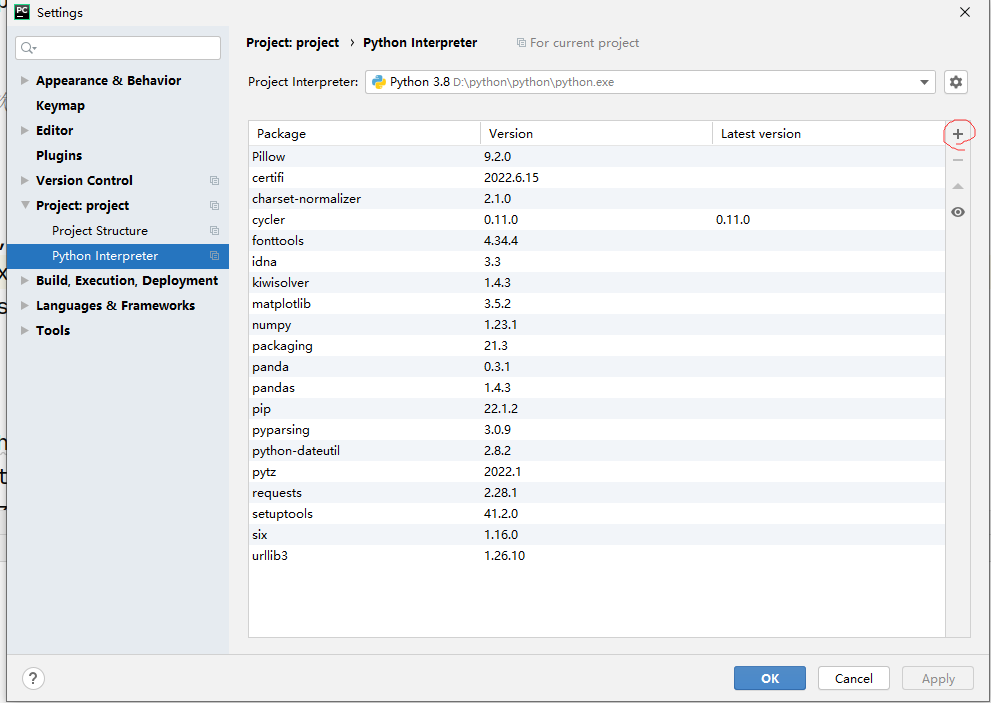在搜索栏中搜索你想安装的包名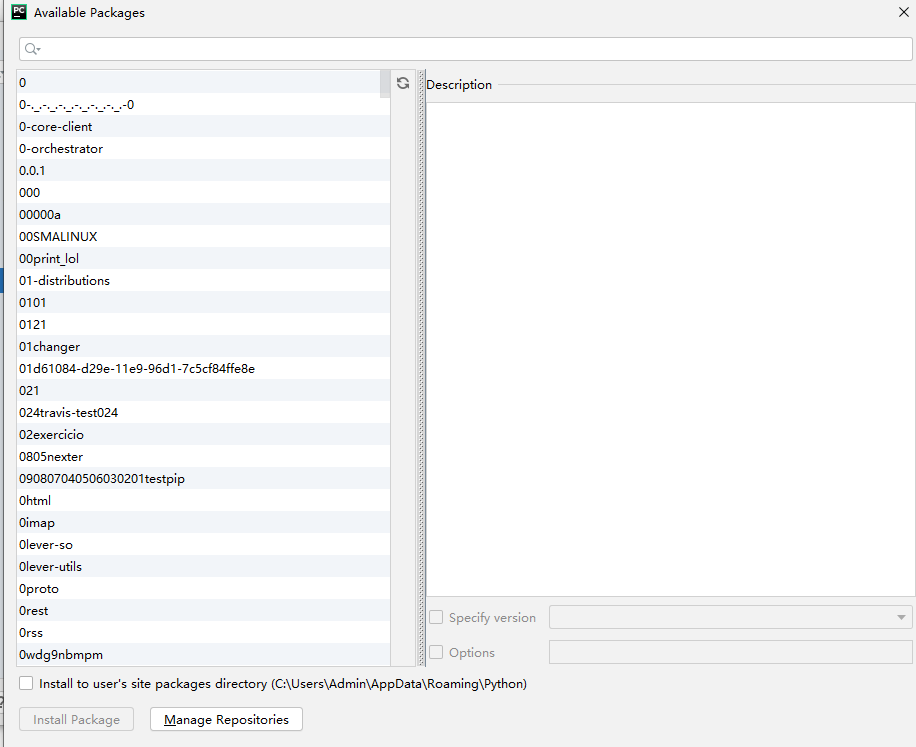点击install Package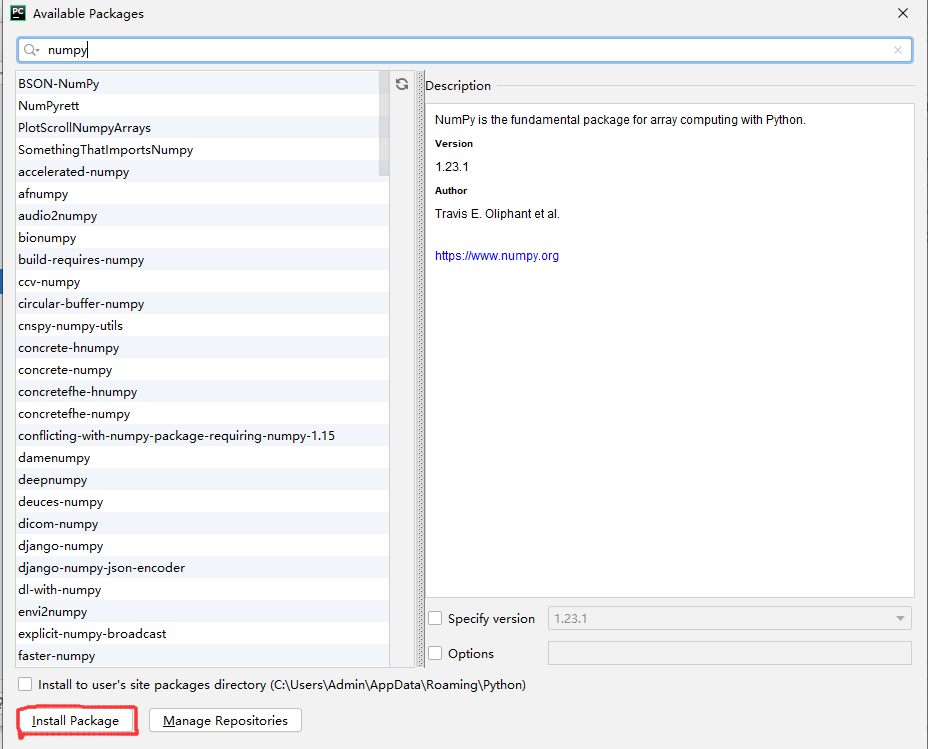上面方法可能会存在的问题：如果搜索不到版本信息，那么可以尝试更新一下pycharm，一般更新到最新版本可能就会成功。

展开全文pycharm matplotlib
• Series：一维数组，与Numpy中的一维array类似。二者与Python基本的数据结构List也很相近，其区别是：List中的元素可以是不同的数据类型，而Array和Series中则只允许存储相同的数据类型，这样可以更有效的使用内存，...
• python学习笔记之pandas导入numpy数组 新手楼主前几天用pandas将CSV文件导入numpy数组的时候，遇到了一个问题，每次首行都会被去掉，后来发现是一个常识性的问题，首行会作为表头不被导入数组，CSV文件如下： 代码...

### python学习笔记之pandas导入numpy数组

新手楼主前几天用pandas将CSV文件导入numpy数组的时候，遇到了一个问题，每次首行都会被去掉，后来发现是一个常识性的问题，首行会作为表头不被导入数组，CSV文件如下：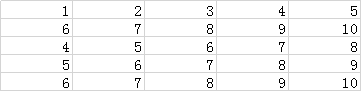代码如下：

import numpy as np
import pandas as pd

zzz = x.loc[:, :].to_numpy()
print(zzz)


结果如图：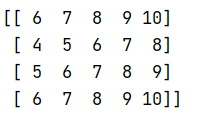为CSV添加表头（首行）之后如下：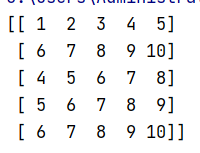新手学习不易，希望能帮到跟我一样被困扰的人。

展开全文python
• 大多数情况下，都是用 NumpyPandas 导入数据。 一、文本文件 1、纯文本文件 filename = 'test.txt' file = open(filename, mode='r') # 以只读方式读取文件 text = file.read() # 读取文件内容 print(file....

大多数情况下，都是用 Numpy 或 Pandas 导入数据。

Pandas 数据框：

• df.head()   # 返回数据框的前几行，默认为5行
• df.tail()   # 返回数据框的后几行，默认为5行
• df.index    # 查看数据框的索引
• df.columns  # 查看数据框的列名
• df.info()   # 查看数据框各列的信息
• data_array = data.values  # 将数据框转换为 Numpy 数组

Numpy 数组：

• data_array.dtype  # 查看数组元素的数据类型
• data_array.shape  # 查看数组维度

# 一、文本文件

## 1、纯文本文件

filename = 'test.txt'
file = open(filename, mode='r')  # 以只读方式读取文件
text = file.read()               # 读取文件内容
print(file.closed)               # 查看文件是否已经关闭
file.close()                     # 关闭文件
print(text)# 关闭已经文件

使用上下文管理器 with：

with open('test.txt', mode='r') as file:
print(file.closed)  # 查看文件是否已经关闭
print(file.closed)      # 关闭已经文件

## 2、表格数据：文本文件

### （1）用 Numpy 导入文本文件

（a）单数据类型文件

import numpy as np

filename = 'iris.txt'
delimiter=',',   # 用于分割各列值的字符
skiprows=2,      # 跳过前两行
usecols=[0, 2],  # 读取并使用第1列和第3列
dtype=str)       # 使用的数据类型
print(data)

（b）多数据类型文件

import numpy as np

filename = 'iris.csv'
# read the first 4 columns
data = np.genfromtxt(filename,
delimiter=',',
names=True,            # 导入时查找列名
usecols=(0, 1, 2, 3))  # 读前四列
print(data.dtype)  # [('sepal_length', '<f8'), ('sepal_width', '<f8'), ('petal_length', '<f8'), ('petal_width', '<f8')]
# read the fifth column
target = np.genfromtxt(filename, delimiter=',', usecols=(4, ), dtype=str)
np.recfromcsv() 函数的 dtype 默认值为 None。

data_array = np.recfromcsv(filename)

### （2）用 Pandas 导入文本文件

filename = 'iris.csv'
nrows=3,         # 读取的行数
sep=',',         # 用于分隔各列的字符
comment='#',     # 用于分割注释的字符
na_values=[""])  # 读取时，哪些值为NA / NaN
print(data)
#    sepal_length  sepal_width  petal_length  petal_width species
# 0           5.1          3.5           1.4          0.2  setosa
# 1           4.9          3.0           1.4          0.2  setosa
# 2           4.7          3.2           1.3          0.2  setosa

# 二、Excel表

file = 'iris.xlsx'
data = pd.ExcelFile(file)
print(data.sheet_names)  # 使用sheet_names属性访问表单名称['iris', 'test']
df_sheet1 = data.parse('iris',          # sheet名字
nrows=3,         # 读取的行数
skiprows=,
names=['sepal_length', 'sepal_width', 'petal_length', 'petal_width', 'species'])
df_sheet2 = data.parse(1,               # # sheet序号
parse_cols=,
skiprows=)
print(df_sheet1)
#    sepal_length  sepal_width  petal_length  petal_width species
# 0           4.9          3.0           1.4          0.2  setosa
# 1           4.7          3.2           1.3          0.2  setosa
# 2           4.6          3.1           1.5          0.2  setosa
print(df_sheet2)
#    1   wang   121424
# 0  2     li  e341515
# 1  3  zhang    53515
# 2  4   tang   532525

# 四、Pickled 文件

import pickle

in_data = [1, 3, 5, 7, 9]
output_file = open("test.pkl", 'wb')
pickle.dump(in_data, output_file)
output_file.close()

input_file = open("test.pkl", 'rb')
print(out_data)  # [1, 3, 5, 7, 9]
# 使用上下文管理器 with：
with open('test.pkl', 'rb') as input_file:
print(out_data)

# 五、HDF5 文件

import h5py
filename = 'test.hdf5'
data = h5py.File(filename, 'r')

# 六、Matlab 文件

import scipy.io
filename = 'workspace.mat'
mat = scipy.io.loadmat(filename)

# 七、SAS 文件

from sas7bdat import SAS7BDAT
with SAS7BDAT('test.sas7bdat') as file:
df_sas = file.to_data_frame()

# 八、Stata 文件

data = pd.read_stata('urbanpop.dta')

# 九、关系型数据库文件

from sqlalchemy import create_engine
engine = create_engine('sqlite://Northwind.sqlite')
table_names = engine.table_names()

查询关系型数据库

con = engine.connect()
rs = con.execute("SELECT * FROM Orders")
df = pd.DataFrame(rs.fetchall())
df.columns = rs.keys()
con.close()

# 使用上下文管理器
with engine.connect() as con:
rs = con.execute("SELECT OrderID FROM Orders")
df = pd.DataFrame(rs.fetchmany(size=5))
df.columns = rs.keys()

使用Pandas 查询关系型数据库

df = pd.read_sql_query("SELECT * FROM Orders", engine)

摘自DataCamp
Learn Python for Data Science Interactively

展开全文python
• import numpy as npimport pandas as pd# 导入数据# 读取csv数据df = pd.read_csv(open(r"D:\Python课程\python数据分析实战习题\4.1 数据导入\4.1\1.csv"))df1 = pd.read_csv(open(r"D:\Python课程\python数据分析...
• numpy是数据处理的基础，pandas也是基于numpy的，首先是numpy数组的创建。 一般我们默认导入了一下库 import numpy as np import pandas as pd 1.numpy创建数组及基础属性 arr=np.array([[1,2,3],[4,5,6],[7,8,9python
•python
• ## python怎么导入pandas

千次阅读 2020-12-03 07:41:17
Pandas 是python的一个数据分析包，最初由AQR Capital Management于2008年4月开发，并于2009年底开源出来，目前由专注于Python数据包开发的PyData开发team继续开发和维护，属于PyData项目的一部分。Pandas最初被作为...
• 下面小编就为大家分享一篇利用numpypandas处理csv文件中的时间方法，具有很好的参考价值，希望对大家有所帮助。一起跟随小编过来看看吧
• import pandas as pd data = pd.read_excel('1.xlsx') 导入excel文件 print(data) data.to_pickle('student.pick') 导出文件 有好多保存文件的形式，可以参考菜鸟教程python 大数据 机器学习
• Pandas最初被作为金融数据分析工具而开发出来，因此，pandas为时间序列分析提供了很好的支持。Pandas的名称来自于面板数据(panel data)和...数据结构：Series：一维数组，与Numpy中的一维array类似。二者与Python...
• 目录Numpy1、概述2、基础操作3、并行化思想4、量化分析应用4.1 索引选取和切片选择4.2 数据转换与规整4.3 逻辑条件进行数据筛选4.4 通用序列函数4.5 文件保存与读取Pandas Numpy 1、概述 Numpy是Python很多科学计算...python
• ## python如何导入pandas

千次阅读 2020-12-04 02:57:41
数据采集链接检查数据 首先...import osimport numpy as npimport pandas as pdimport matplotlib.pyplot as plt%matplotlib inline现在开始运行，使用Pandas把数据加载到数据帧里，并且使用“head”函数显示前几行...
• 几个简单步骤即可顺利安装 pygame, pandas, numpy和scipy等软件包。python scipy pygame
• 1、Python数据科学速查表 - Numpy 基础 2、Python数据科学速查表 - Pandas 基础 3、Python数据科学速查表 - Pandas 进阶 4、Python数据科学速查表 - Matplotlib 5、Python数据科学速查表 - Jupyter Notebook
• python导入pandas具体步骤方法，Pandas最初被作为金融数据分析工具而开发出来，因此，pandas为时间序列分析提供了很好的支持。Pandas的名称来自于面板数据(panel data)和python数据分析(data analysis)。panel data...
• 报错内容Import "pandas" could not be resolved from source import pandas as pd import numpy as np 思考大概可能发生的问题 一、想在vscode中引用python的包，经过查看安装文件发现没有问题，此时可卸载重新...vscode python
• 尝试使用 import pandas 导入pandas时，我不断收到此消息 .文件“C：\ Users \ Seif \ AppData \ Local \ Programs \ Python \ Python36-32 \ lib \ site-packages \ pandas__init __ . py”，第35...
• 历史数据通常会以CSV的格式来存储，或者能够方便地转化为CSV格式。CSV 文件是用逗号分隔的文本文件。...数据导入时，设置字段属性名称，有助于提高数据处理程序的可读性。 （2）文件中的注释 在 CSV ...
• 很多库在使用pip安装后，会与numpy的版本产生冲突，一般都是numpy的版本低导致的，使用命令进行更新即可。此命令默认更新成的都是符合本机要求的numpy最新版本。如果在线安装或升级过慢，可以先将whl文件下载下来...python
• 我在vscode下载jupyter拓展，但是导入不了pandas库 我想用vscode的jupyter导入pandaspython jupyter
• 随机变量分为连续的和离散的两种 import pandas as pd #导入pandas包，命名为pd import numpy as np #导入numpy包，命名为np 执行结果呈现：（下面的文章，代码在上部分，执行结果在下部分） 数据预处理，处理缺失值...python 数据挖掘
• pandas库跟numpy库都是python之中的用于数据分析的非常好用的库，但numpy处理的文件有限，只能处理只能读取txt文件和csv文件，在处理较多数据时我们一般用pandas库从excel中读取数据。 目录前言一.numpy库的的文件...
• pandas：是在numpy的基础上除了帮我们处理数据型的数组，还能帮助我们处理字符串，列表，以及时间序列等等所有数据类型都是可以处理的 一，数据分析是用适当的方法对收集来的大量数据进行分析.（帮助人们做出判断...python 数据挖掘...